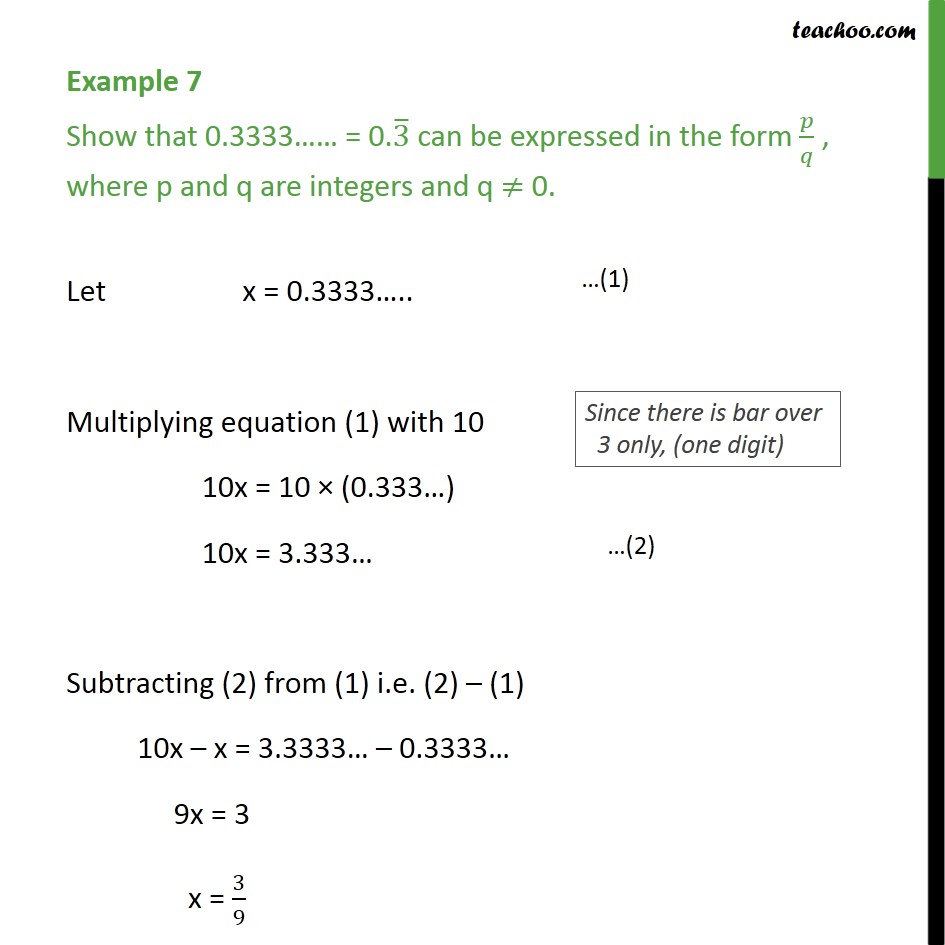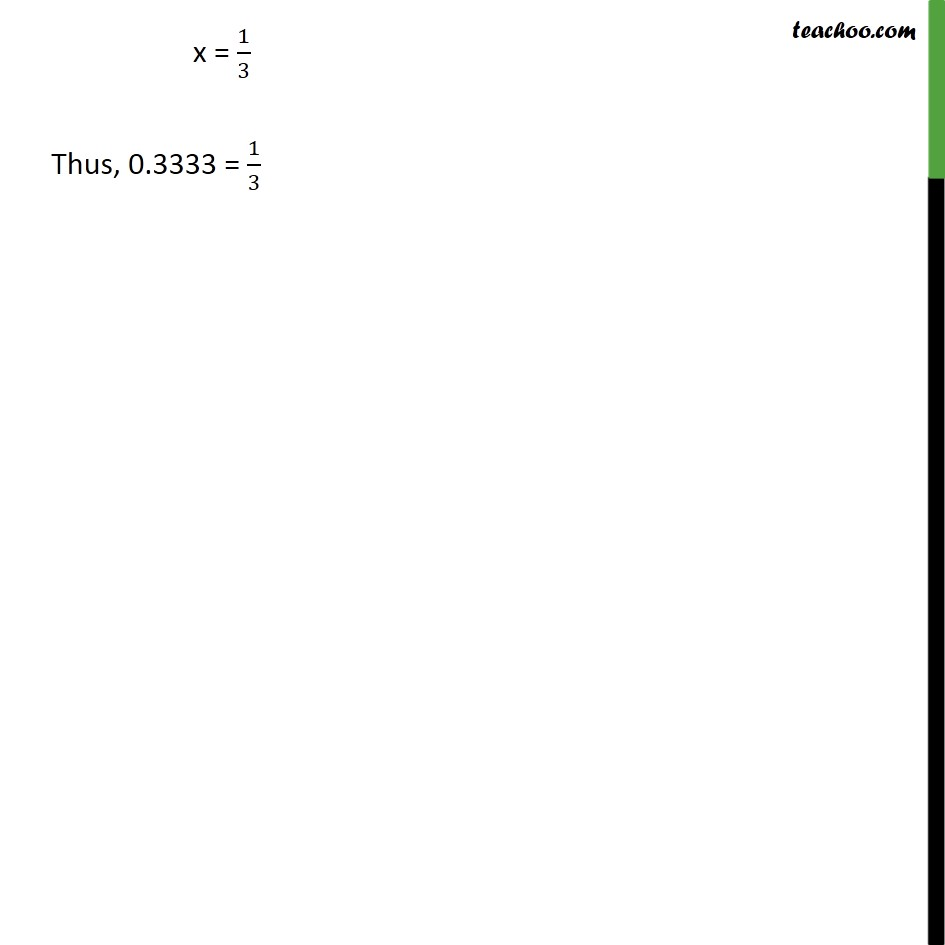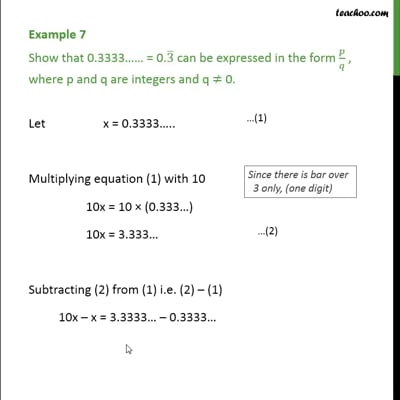Examples

Chapter 1 Class 9 Number Systems
Serial order wiseThis video is only available for Teachoo black users

Maths Crash Course - Live lectures + all videos + Real time Doubt solving!

### Transcript

Example 7 Show that 0.3333 = 0.3 can be expressed in the form / , where p and q are integers and q 0. Let x = 0.3333 .. Multiplying equation (1) with 10 10x = 10 (0.333 ) 10x = 3.333 Subtracting (2) from (1) i.e. (2) (1) 10x x = 3.3333 0.3333 9x = 3 x = 3/9 x = 1/3 Thus, 0.3333 = 1/3